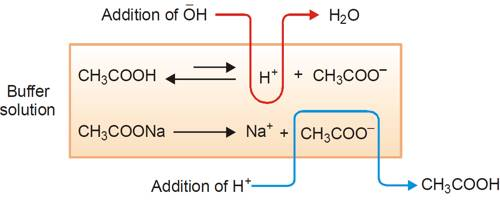Chemistry

# Mechanism of Buffer ActionMechanism of buffer action

Buffer Action: The property of the solution to resist the changes in its pH value on the addition of small amounts of strong acid or base is known as buffer action”.

A buffer is a solution containing a weak acid and its conjugate base in similar amounts. The way in which a buffer solution acts may be illustrated by considering a buffer solution made up of CH3COOH and CH3COONa. A buffer solution may be prepared by mixing comparable molar amounts of ethanoic acid and sodium ethanoate in water. In the solution we have the following situation:

CH3COOH (aq) ↔ CH3COO (aq) + H+ (aq)

CH3COONa (aq) → CH3COO (aq) + Na+ (aq)

The mechanism of buffer action can be understood by considering an acidic buffer made of a weak acid like Acetic acid and its sodium salt Sodium acetate. Sodium acetate, however, exists as acetate ions and Na+ ions.

The salt exists completely as ions.Thus the buffer contains both acid (CH3COOH) and its conjugate base (CH3COO). When a small quantity of an acid is added the hydrogen ions are removed by the conjugate base (CH3COO) as follows:

H+ (aq) + CH3COO (aq) ↔ CH3COOH (aq)

Since ethanoic acid is only slightly dissociated and in the form CH3COOH, it does not contribute any H+ ion, the pH of the resulting solution will remain practically constant. Due to the removal of most of the added H+, there is no appreciable decrease in pH. The reaction nearly goes to completion because CH3COOH is a weak acid and its ions have a strong tendency to form non-ionized CH3COOH molecules. If, however, a strong base is added, the added OH ion is neutralized by the reaction with the acid in the buffer,

CH3COOH (aq) + OH (aq) → CH3COO (aq) + H2O (l)

One may also consider that the added OH- ion reacts with the H+ ion to produce water. The added OH- ions are removed and the acid equilibrium shifts to the right to replace the H+ ions used up. Thus, there is a very slight change in the pH value. Now, if you add a drop of NaOH, the OH ions react with the free acid to give undissociated water molecules.

Since additional OH ions of the base are consumed or neutralized, the pH of the solution remains unchanged. This resistance to change in pH on addition of base is called as reserve acidity and is due to CH3COOH. The effect is the neutralization of most of the added OH ions by CH3COOH. Therefore, there is no appreciable change in pH. When a strong base is added, the acid present in the buffer neutralizes the hydroxide ions (OH-)

In either case, the effect of the added acid or base is effectively balanced so that the pH of the solution remains approximately constant. Similarly, the mechanism of basic buffers can be explained.

So, the weak acid and weak base remain in the solution with high concentrations since they only rarely react with the water. However, they are very likely to react with any added strong base or strong acid.

Characteristics of Buffer: A buffer resists changes in pH by having a relatively high concentration of acid or base, or, most often, both an acid and a base.

• It has a definite pH value.
• Its pH value doesn’t change on keeping for a long time.
• Its pH value doesn’t change on dilution.
• Its pH value doesn’t change even with the addition of a small amount of a strong acid or a base.